# RF Sheet Resistance

This page will show you how to calculate sheet resistance of a layer of metal versus frequency. By the way, what we call "RF sheet resistance" is often called "surface resistance" or "surface impedance" or other names in textbooks.

### DC sheet resistance

The term sheet resistance should be familiar if you work with electronic thin films. It is a function of the bulk resistivity of a metallic film, and its thickness. We discuss it further on this page. Sheet resistance, Rsh, is given in ohms per square, where squares are the unitless dimension of length divided by width.

For a more complete discussion of direct current sheet resistance, go to our page on this topic. In summary, for direct current, the sheet resistance is calculated from the metal resistivity and thickness: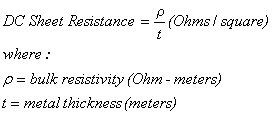### Sheet resistance and sheet conductance as functions of frequency

Something that is not often considered is that sheet resistance is a function of frequency. Applied in the RF world, errors can result from using the DC definition.

The assumption of constant sheet resistance is only valid for conductors that are thin compared to skin depth (which is often the case for thin-film resistors, but never the case for transmission lines.)

Knowing RF sheet resistance versus frequency of interconnect metals (copper or gold for examples) can be a very useful short-cut for evaluating attenuation of strip conductors such as microstrip.

We offer a free download that you should grab if you want to analyze RF sheet resistance of various metals (including stacked metals) that you specify. Look for it here. It's near the bottom of the page.

#### Calculating maximum sheet conductance (minimum sheet resistance)

Sheet conductivity is the inverse of sheet resistance, its units are Siemen-squares, or mho-squares. This quantity is useful when you are dealing with multiple-layer conductors, as their conductivities can be added in parallel, then the sum can be re-inverted and expressed in a composite sheet resistance. When RF is involved, the so called skin-effect can become apparent, and therefore DC calculations of conductivity and resistivity are invalid. The skin effect is taken into account by using a decreasing exponential factor whose exponent is inversely proportional to a parameter called skin depth.

Maximum sheet conductance is the best you can do, and is a function of frequency. Adding more metal beyond five skin depths doesn't help!

The percentage of conduction that is achieved versus depth into a metal varies as the negative exponential of the depth expressed in skin depths. At the surface, complete conduction takes place, and the resistivity of the metal is 100% of its value at DC, equal to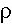. At one skin depth, the metal's conductivity has been reduced to 36.8% of, at 2 skin depths, 13.5%, etc. By the time you reach five skin depths the metal's conductivity is reduced to just 0.7% of its full value. That is where the rule of thumb of five skin depths comes from, adding tons of additional metal beyond five skin depths can only reduce your RF resistance by 0.7%, so why bother? Expressed below is what we call the incremental RF conductivity, it is the conductivity at a given depth, reduced by the skin depth equation: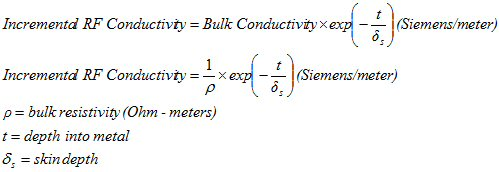Thanks for the correction, Ron! And thanks to Giovanni, our use of the Greek alphabet is now consistent for skin depth! If we integrate all of the conductivity of the thin film from the surface to include infinite skin depths, we arrive at the maximum sheet conductance for a given frequency. This is different from DC sheet conductivity, which can be quite a bit higher since every free electron in the metal contributes to conduction during direct current. Although we have pledged never to use calculus on this web site, integrating an exponential function is so easy that even we can do it (but we won't show you all of the intermediate steps that required a big eraser). The maximum sheet conductance is: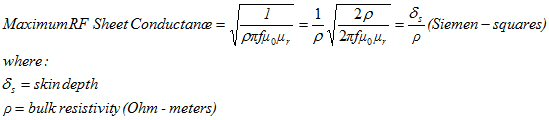(Thanks for the correction, Michael!) The maximum RF sheet conductance is in units of Seimen-squares (or mho-squares) which is the inverse of sheet resistance (units of ohms/square). Similarly, the minimum RF sheet resistance is just the reciprocal of the above equation: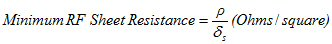Voila! that equation looks an awful lot like the DC sheet resistance equation (top of page) except the skin depth is now in the dominator instead of the conductor's thickness.

Remember, this is the best you can achieve, no matter how much more metal you add to the transmission line! Now let's look at what this means for various metals. Click here to look up conductivities of various metals. The plot below compares aluminum, gold, copper and silver. Silver is best, followed by copper, then gold, then aluminum. At DC you can achieve nearly zero sheet resistance, because the skin depth is infinite. But to get truly zero sheet resistance, you'd need infinite metal thickness! The minimum sheet resistance will have an effect on the transmission line loss, which you can read about on this page.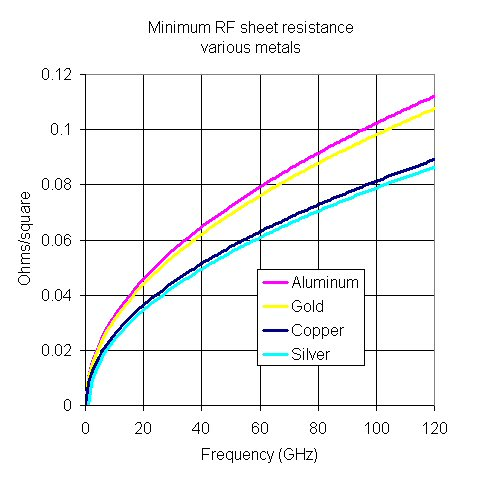In any case, how about a rule of thumb?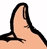The minimum RF sheet resistance you can achieve is on the order of 30 milli-ohms/square at X-band, and increases as the square-root of frequency up to 100 milliohms/square at W-band. The exact value will depend on the metal's conductivity.

### Examples

Here's some example RF sheet resistance calculations.

Here's the special case of high permeability metals.

Author : Unknown Editor